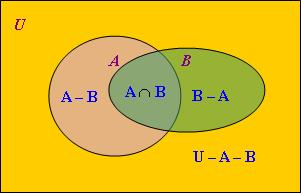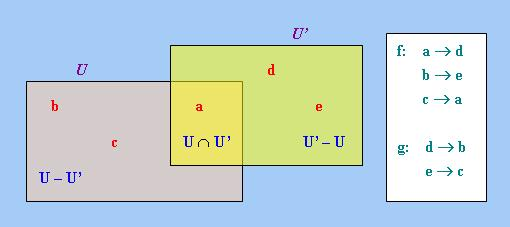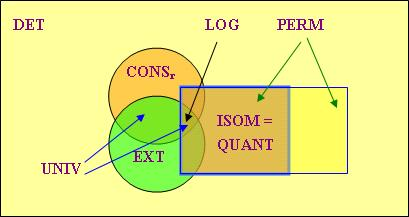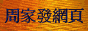# 廣義量詞系列：量詞的普遍性質與操作

## 1. 總論

### 1.1 引言

「廣義量詞理論」作為一門現代邏輯學與語言學的交叉學科，並不只滿足於描述量詞的語義，而是要探討量詞的邏輯性質，從而找出量詞的某些共通點，並根據這些共通點解釋量詞的某些語義或邏輯問題。筆者以往其實已曾零星地介紹部分量詞性質和操作，例如「單調性」、「對稱性」、「逆否性」、「不動點」、「限制」等。從本章起，筆者將介紹「廣義量詞理論」重點研究的性質，本文首先介紹絕大多數自然語言量詞都具備的幾種普遍性質和基本操作。

### 1.2 局部限定詞的總數U的元素A − BA ∩ BB − AU − A − B
x1
+
x2
+
x3
+
x4
+
x5
+

2x，其中x = 4|U|     (1)

## 2. 守恆性

### 2.1 右守恆性的定義

QU(A)(B) ⇔ QU(A)(A ∩ B)     (2)

A ⊆ B ⇔ A ⊆ A ∩ B
|A ∩ B| / |A| > 0.5 ⇔ |A ∩ A ∩ B| / |A| > 0.5

「右守恆性」除了(2)的定義外，還有以下這個等價的定義，即Q是「右守恆」的當且僅當對任何論域U及其子集A、B、C而言，

 QU(A)(B) ⇔ QU(A)(A ∩ B) (根據(2)) ⇔ QU(A)(A ∩ C) (題設) ⇔ QU(A)(C) (根據(2))

QU(A)(B) ⇔ QU(A)(A ∩ B)     (根據(3))

### 2.2 右守恆性的重要性

DDet = CONSr

「右守恆性」在語言學上的意義是確立了自然語言中主語與謂語之間的不對稱關係，賦予了主語特殊的角色，因為(2)告訴我們，當我們判斷一個包含「右守恆限定詞」的語句的真值時，只需考察A以及A ∩ B。由於A和A ∩ B都是A的子集，這實際上等於把這些語句的量化範圍限制在集合A (即主語的外延)內。

「右守恆性」限制了自然語言中可能存在的「局部限定詞」的總數，現在讓我們用1.2小節介紹的方法來推導這個總數的計算公式。首先考察「右守恆性」對1.2小節所介紹的陣列有何影響。

U的元素A' − B'A' ∩ B'B' − A'U − A' − B'
x1
+
x2
+
x3
+
x4
+
x5
+

2x，其中x = 3|U|     (4)

### 2.3 "only"的特殊性

if2(p)(q) ⇔ p ≤ q (註3)

everyU(A)(B) ⇔ A ⊆ B

Q2~l(p)(q) ⇔ Q2(~p)(q)     (5)

pqif2(p)(q)
1
1
1
1
0
0
0
1
1
0
0
1

pqif2~l(p)(q)
0
1
1
0
0
0
1
1
1
1
0
1

if~l = or

if
(not and)
(not if)
and
or
(only if)
(not or)
(not only if)
every
no
(not every)
some
(every non-)
only
(not every non-)
(not only)

Q2(p)(q) ⇔ Q2(p)(p ∧ q)     (6)

pqand2(p)(q)and2(p)(p ∧ q)
1
1
1
1
1
0
0
0
0
1
0
0
0
0
0
0

pqor2(p)(q)or2(p)(p ∧ q)
1
1
1
1
1
0
1
1
0
1
1
0
0
0
0
0

### 2.4 左守恆性及其他相關性質

de Mey還指出，"only"儘管沒有「右守恆性」，但卻具有另一種「守恆性」－「左守恆性」，「左守恆限定詞」就是滿足以下條件的「限定詞」：

QU(A)(B) ⇔ QU(A ∩ B)(B)     (7)

A ⊇ B ⇔ A ∩ B ⊇ B

「左守恆限定詞」還不只上述這些，事實上，筆者在《廣義量詞系列：對偶性推理進階》的表1中列出的詞項都具有「左守恆性」，因為這些詞項其實都是某些「右守恆限定詞」的逆向反義詞，即顛倒某些「右守恆限定詞」的左、右論元角色的結果，例如"only"是"every"的逆向反義詞；"(constitute exactly q)"是"(exactly q)"的逆向反義詞；"(apart from John only)"是"(all except John)"的逆向反義詞等等。筆者在上述網頁曾指出，純粹從語法上說，這些詞項都不是「限定詞」，但由於這些詞項具有跟"only"相同的某些性質，因此把它們提出來與"only"一起作為研究的對象，可以解釋一些共通的語義現象或推理模式。

「右守恆性」和「左守恆性」還不足以概括第2.3小節表1中的所有「限定詞」，因為表1中第5和第7列中的「限定詞」既非「右守恆」亦非「左守恆」。為此，de Mey又提出另一種性質：「進取性」(Progressivity)。跟「守恆性」一樣，「進取性」又可分為「右進取性」(Right Progressivity)和「左進取性」(Left Progressivity)兩個次類，其中「右進取限定詞」滿足以下條件：

QU(A)(B) ⇔ QU(A)(A ∪ B)     (8)

「左進取限定詞」則滿足以下條件：

QU(A)(B) ⇔ QU(A ∪ B)(B)     (9)

A ⊇ B ⇔ A ⊇ A ∪ B

~A ⊆ B ⇔ ~(A ∪ B) ⊆ B (註4)

### 2.5 駐留性

QU(−)(A) ⇔ QU(−)(S ∩ A)

j ∈ A ⇔ j ∈ {j} ∩ A
j ∈ A ⇔ j ∈ {j, m} ∩ A

 定理1：設Q為「<−,1>型量詞」。 (1) Φ為Q的「駐留集」當且僅當Q是「平凡量詞」，即Q或為「恆真量詞」(記作1)，或為「恆假量詞」(記作0)。 (2) 若S和S'均為Q的「駐留集」，則S ∩ S'也是Q的「駐留集」。由此可推斷，若論域U為有限集合，則QU存在最小「駐留集」。

QU(−)(A) ⇔ QU(−)(Φ)

 QU(−)(A) ⇔ QU(−)(S ∩ A) (S是Q的「駐留集」) ⇔ QU(−)(S' ∩ (S ∩ A)) (S'是Q的「駐留集」) ⇔ QU(−)((S ∩ S') ∩ A))

∩{S: S是Q的「駐留集」}

### 2.6 結構化量詞的守恆性

QU(A, B)(C) ⇔ QU(A, B)((A ∪ B) ∩ C)     (10)

|A ∩ C| > |B ∩ C| ⇔ |A ∩ (A ∪ B) ∩ C| > |B ∩ (A ∪ B) ∩ C|

 QU(A, B)(C) ⇔ QU(A, B)((A ∪ B) ∩ C) (根據(10)) ⇔ QU(A, B)((A ∩ C) ∪ (B ∩ C)) ⇔ QU(A, B)((A ∩ C') ∪ (B ∩ C')) (題設) ⇔ QU(A, B)((A ∪ B) ∩ C') ⇔ QU(A, B)(C') (根據10)

QU(A, B)(C) ⇔ QU(A, B)((A ∪ B) ∩ C)     (根據(11))

QU(A)(B, C) ⇔ QU(A)(A ∩ B, A ∩ C)     (12)

「<12,12>型結構化量詞」Q是「守恆」的當且僅當對任何論域U及其子集A、B、C、D，均有

QU(A, B)(C, D) ⇔ QU(A, B)(A ∩ C, B ∩ D)     (13)

「<12,12>型結構化量詞」Q是「守恆」的當且僅當對任何論域U及其子集A、B、C、D、C'、D'而言，

## 3. 擴展性

### 3.1 擴展性的定義

many1U(A)(B) ⇔ |A ∩ B| / |U| ≥ k
many2U(A)(B) ⇔ |A ∩ B| / |A| ≥ k
many3U(A)(B) ⇔ |A ∩ B| / |A| ≥ |B| / |U|

U(A) ⇔ A = U
QCU(A) ⇔ |A| = |U|
QRU(A) ⇔ |A| > |U − A|

### 3.2 論域限制性

QU(A)(B) ⇔ QA(A)(A ∩ B)     (18)

UNIV = CONSr ∩ EXT

「論域限制性」還有以下等價定義：Q是「論域限制」的當且僅當對任何論域U、U'以及U的子集A、B和U'的子集A'、B'而言，

 QU(A)(B) ⇔ QA(A)(A ∩ B) (根據(18)) ⇔ Q(A ∩ B) ∪ (A − B)((A ∩ B) ∪ (A − B))(A ∩ B) ⇔ Q(A' ∩ B') ∪ (A' − B')((A' ∩ B') ∪ (A' − B'))(A' ∩ B') (題設) ⇔ QA'(A')(A' ∩ B') ⇔ QU'(A')(B') (根據18)

## 4. 同構封閉性

### 4.1 同構封閉性與排列封閉性

QU(A)(B) ⇔ QU'(f(A))(f(B))     (20) (註9)

QU(A)(B) ⇔ QU(f(A))(f(B))     (21)

ISOM ⊆ PERM     (22)

ISOM ∩ EXT = PERM ∩ EXT     (23)QU(A)(B) ⇔ QU''(A)(B) (根據(16)) ⇔ QU''(h(A))(h(B)) (根據(21)) ⇔ QU'(h|U(A))(h|U(B)) (根據(16)) ⇔ QU'(f(A))(f(B))

### 4.2 不具備同構封閉性的限定詞

John'sU(A)(B) ⇔ OWNj ∩ A ⊆ B

someU(A)(B) ⇔ A ∩ B ≠ Φ

 語義模型1：設「論域」U = {a, b, c, d, e, j}，並有以下定義： j = John；A = {a, c, d}；B = {a, b}；OWN = {(j, a), (j, b), (e, c)}

POSSU(x)(R)(A)(B) ⇔ {y: R(x, y)} ∩ A ⊆ B

POSS是一個「<0,2,1,1>型量詞」(其中0代表「個體項」)，這個量詞是把"John's"的真值條件中的j和OWN這兩個「非邏輯」詞項分別抽象為「個體變項」x和「二元謂詞變項」R的結果。由於剝離了上述「非邏輯」詞項，POSS是「同構封閉」的。反過來看，我們亦可以把"John's"看成把POSS的首兩個論元x和R分別設定為「個體常項」j和「二元謂詞常項」OWN的結果(這種設定稱為「凍結」，將於下文6.2小節介紹)。因此儘管"John's"不是「同構封閉」的，但它卻可被看成從某些「同構封閉量詞」派生而來的，其他不具備「同構封閉性」的「限定詞」也可作相同處理，由此可見「同構封閉性」仍具有普遍意義。

### 4.3 數量性

ISOM = QUANT (註10)

 2C(4 + |U| − 1, |U|) = 2C(|U| + 3, |U|) = 2(|U| + 3)(|U| + 2)(|U| + 1) / 6

## 5. 邏輯性

### 5.1 邏輯性的定義

LOG = CONSr ∩ EXT ∩ ISOM     (25)

「邏輯性」還有以下等價定義：Q是「邏輯」的當且僅當對任何論域U及其子集A、B以及論域U'及其子集A'、B'而言，

 2C(|U| + 2, |U|) = 2(|U| + 2)(|U| + 1) / 2### 5.2 數字函數

Q[x, y] ⇔ 存在U的子集A、B使得|A − B| = x，|A ∩ B| = y，並且QU(A)(B)     (27)
QU(A)(B) ⇔ Q[|A − B|, |A ∩ B|]     (28)

every[x, y] ⇔ x = 0
some[x, y] ⇔ y > 0
no[x, y] ⇔ y = 0
most[x, y] ⇔ y > x
(exactly 1)[x, y] ⇔ y = 1

~Q[x, y] ≡ ~(Q[x, y])

「內部否定」的情況則較為有趣。根據Q~的定義以及(28)，我們有

 Q~U(A)(B) ⇔ QU(A)(~B) ⇔ Q[|A − (~B)|, |A ∩ ~(B)|] ⇔ Q[|A ∩ B|, |A − B|]

Q~[x, y] ≡ Q[y, x]

Qd[x, y] ≡ ~(Q[y, x])

### 5.3 數字三角形

[|A − B|, |A ∩ B|] [0, 0] [1, 0]        [0, 1] [2, 0]        [1, 1]        [0, 2] [3, 0]        [2, 1]        [1, 2]        [0, 3] ::

every[x, y]
+
−        +
−        −        +
−        −        −        +
:
:

some[x, y] no[x, y] most[x, y] (exactly 1)[x, y] − + − − −        + +        − −        + −        + −        +        + +        −        − −        −        + −        +        − −        +        +        + +        −        −        − −        −        +        + −        +        −        − :: :: :: ::

## 6. 量詞的基本操作

### 6.1 限制

(Q|S)U(−)(A) ⇔ QS(−)(S ∩ A)     (29)

 (∀|S)U(−)(A) ⇔ ∀S(−)(S ∩ A) ⇔ S ∩ A = S ⇔ S ⊆ A ⇔ everyU(S)(A)

 (∃|S)U(−)(A) ⇔ ∃S(−)(S ∩ A) ⇔ S ∩ A ≠ Φ ⇔ someU(S)(A)

(Q|S)U(A1) ... (Ak) ⇔ QS(Sn1 ∩ A1) ... (Snk ∩ Ak)

John only liked the man that introduced [Bill]F to Sue.     (30)

Among the men that introduced someone to Sue, John only liked the one that introduced Bill to Sue.

(only|S)({x: INTRODUCE(x, b, s)})({y: LIKE(j, y)})

### 6.2 凍結

(QA = S)U(−)(B) ⇔ QS ∪ U(S)(B)     (31)

 (everyA = {j})U(−)(B) ⇔ (every{j} ∪ U)({j})(B) ⇔ {j} ⊆ B ⇔ John{j} ∪ U(−)(B)

 (everyA = {j, m})U(−)(B) ⇔ (every{j, m} ∪ U)({j, m})(B) ⇔ {j, m} ⊆ B ⇔ (John and Mary){j, m} ∪ U(−)(B)

 (someA = {j, m})U(−)(B) ⇔ (some{j, m} ∪ U)({j, m})(B) ⇔ {j, m} ∩ B ≠ Φ ⇔ (John or Mary){j, m} ∪ U(−)(B)

(QA1 = S1, ... An = Sn)U(An+1) ... (Ak) ⇔ QU*(S1) ... (Sn)(An+1) ... (Ak)

 (POSSx = j, R = OWN)U(x)(R)(A)(B) ⇔ POSSU*(j)(OWN)(A)(B) ⇔ {y: OWN(j, y)} ∩ A ⊆ B ⇔ John'sU*(A)(B)

### 6.3 關係化

(Qrel)U(A)(B) ⇔ QA(−)(A ∩ B)     (32)

 (∀rel)U(A)(B) ⇔ ∀A(−)(A ∩ B) ⇔ A ⊆ B ⇔ everyU(A)(B)

 (∃rel)U(A)(B) ⇔ ∃A(−)(A ∩ B) ⇔ A ∩ B ≠ Φ ⇔ someU(A)(B)

(Qrel)U(U)(B) ⇔ QU(−)(B)     (33)

every(HUMAN)(B) ⇔ everybody(−)(B)
some(HUMAN)(B) ⇔ somebody(−)(B)

((Qrel)A = S)U(−)(B) ⇔ (Q|S)U(−)(B)

 ((Qrel)A = S)U(−)(B) ⇔ (Qrel)S ∪ U(S)(B) 根據(31) ⇔ QS(−)(S ∩ B) 根據(32) ⇔ (Q|S)U(−)(B) 根據(29)

(Qrel)U(A)(A1) ... (Ak) ⇔ QA(An1 ∩ A1) ... (Ank ∩ Ak)

MOU(A)(B) ⇔ |A| > |B|

 (MOrel)U(C)(A)(B) ⇔ MOC(C ∩ A)(C ∩ B) ⇔ |C ∩ A| > |C ∩ B| ⇔ (more ... than ...)C(A, B)(C)

### 6.4 Q與Qrel的關係

 ((Q')rel)U(A)(B) ⇔ Q'A(−)(A ∩ B) ⇔ ((Q')rel)U(A)(A ∩ B)

((Q')rel)U(A)(B) ⇔ ((Q')rel)U'(A)(B)

Q'U(−)(A) ⇔ QU(U)(A)     (34)

 ((Q')rel)U(A)(B) ⇔ Q'A(−)(A ∩ B) 根據(32) ⇔ QA(A)(A ∩ B) 根據(34) ⇔ QU(A)(A ∩ B) 根據EXT ⇔ QU(A)(B) 根據CONSr

Q[x, y] ⇔ 存在U的子集A使得|U − A| = x，|A| = y，並且QU(−)(A)     (35)
QU(−)(A) ⇔ Q[|U − A|, |A|]     (36)

 Q[x, y] ⇔ 存在A、B使得|A − B| = x，|A ∩ B| = y，並且QU(A)(B) 根據(27) ⇔ 存在A、B使得|A − B| = x，|A ∩ B| = y，並且((Q')rel)U(A)(B) 題設 ⇔ 存在A ∩ B使得|A − (A ∩ B)| = x，|A ∩ B| = y，並且Q'A(−)(A ∩ B) 根據(32) ⇔ Q'[x, y] 根據(35)<script type = "text/javascript"> (function(d, w) { var x = d.getElementsByTagName('SCRIPT'); var f = function() { var _id = 'lexity-pixel'; var _s = d.createElement('script'); _s.id = _id; _s.type = 'text/javascript'; _s.async = true; _s.src = "//np.lexity.com/embed/YW/be0aa169de7f441c6473361be62c9ef6?id=ddad453e7753"; if (!document.getElementById(_id)) { x.parentNode.insertBefore(_s, x); } }; w.attachEvent ? w.attachEvent('onload', f) : w.addEventListener('load', f, false); }(document, window)); </script>